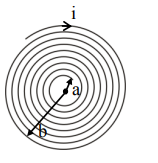# A coil having N turns is wound tightly in the form of a spiral`
Question:

A coil having $N$ turns is wound tightly in the form of a spiral with inner and outer radii 'a' and 'b' respectively. Find the magnetic field at centre, when a current I passes through coil:

1. $\frac{\mu_{0} I N}{2(b-a)} \log _{e}\left(\frac{b}{a}\right)$

2. $\frac{\mu_{0} \mathrm{I}}{8}\left[\frac{a+b}{a-b}\right]$

3. $\frac{\mu_{0} I}{4(a-b)}\left[\frac{1}{a}-\frac{1}{b}\right]$

4. $\frac{\mu_{0} I}{8}\left(\frac{a-b}{a+b}\right)$

Correct Option: 1

Solution:No. of turns in $\mathrm{dx}$ width $=\frac{\mathrm{N}}{\mathrm{b}-\mathrm{a}} \mathrm{dx}$

$\int d B=\int_{a}^{b}\left(\frac{N}{b-a}\right) d x \frac{\mu_{0} i}{2 x}$

$\mathrm{B}=\frac{\mathrm{N} \mu_{0} \mathrm{i}}{2(\mathrm{~b}-\mathrm{a})} \ell \mathrm{n}\left(\frac{\mathrm{b}}{\mathrm{a}}\right)$

Option (1)